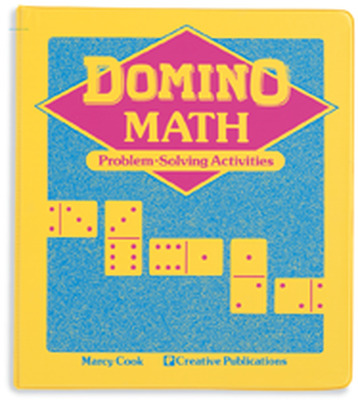# Domino Math

Each reproducible lesson provides a series of math practice problems to be solved with a single set of Double-Six Dominoes (sold separately).Students compute the problems again and again while exploring triangles, squares, paths, bridges, matrices, and more.

Sort by: